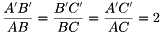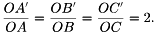# Task 5 – Distance from Point of Similarity

In the figure below, \$latex \triangle ABC \sim A’B’C’\$ and point \$latex P\$ is the point of similarity of the two triangles.

1.) What is the ratio of the lengths of the corresponding sides?

2.) What is the ratio of the distance from point P to the corresponding vertices?

3.) What conjecture can you make from your answer in 1 and 2? Verify your conjectures by drawing a different pair of similar triangles.

Explanation

The ratio of the corresponding sides of two similar triangles is the same as the ratio of the distance of their corresponding vertices to the center of similarity. The ratio of the corresponding sides of two similar triangles is the same as the ratio of the distance of their corresponding vertices. In the figure above, ∆ABC ~ ∆A’B’C’ soand